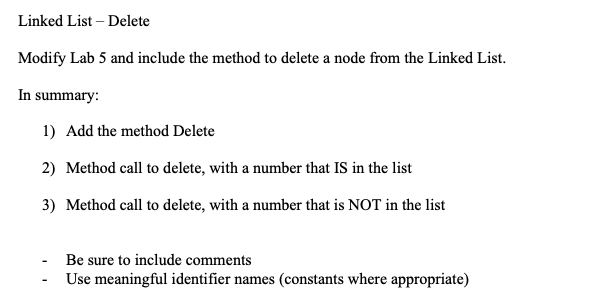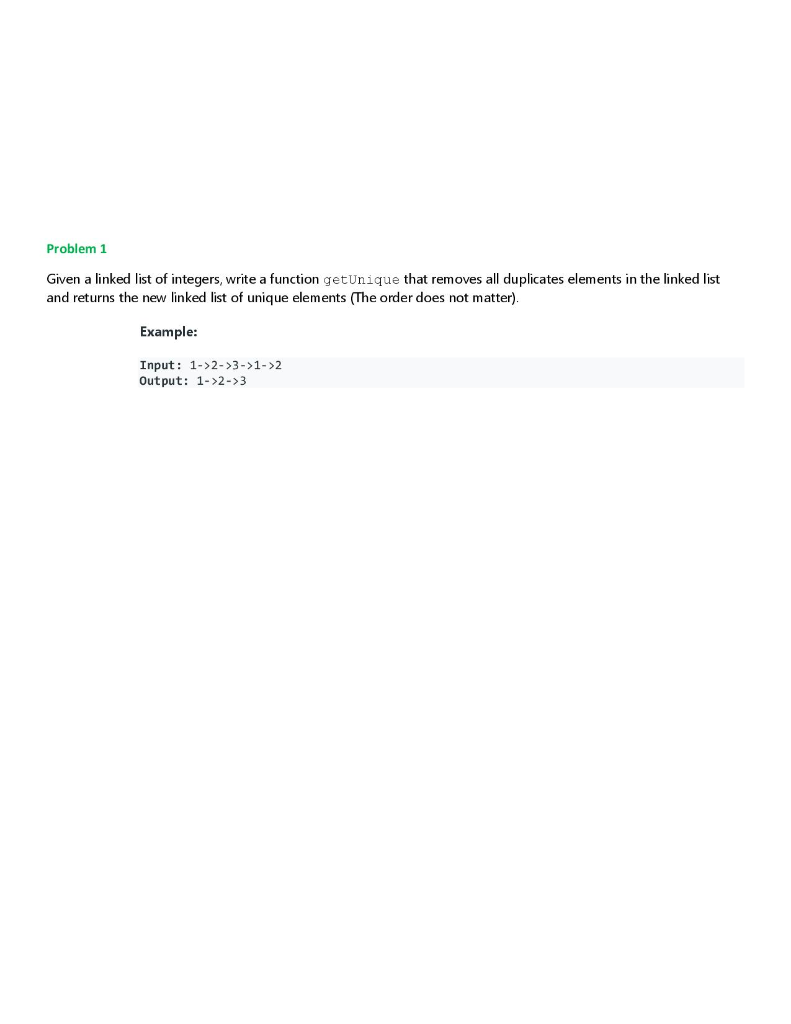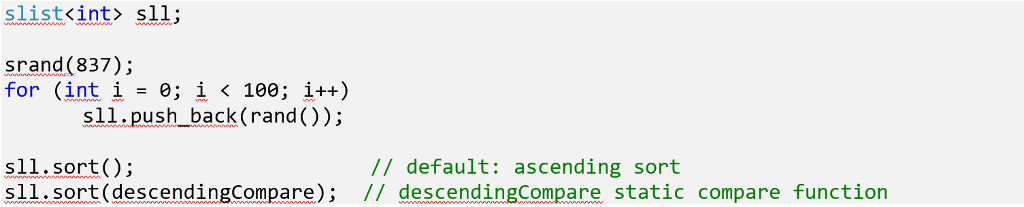Question

# Doubly Linked List The assignment is to modify the below code in any way (like changing the method of a function). Time...

The assignment is to modify the below code in any way (like changing the method of a function). Time complexity is omitted. Any methods/functions below could be changed into something different.

I was thinking of changing the method of getting size of list and maybe change from numbers to letters for nodes.

1. `import java.util.Scanner;`
2. ```
```
3. `/*  Class Node  */`
4. `class Node`
5. `{`
6. `    protected int data;`
7. `    protected Node next, prev;`
8. ```
```
9. `    /* Constructor */`
10. `    public Node()`
11. `    {`
12. `        next = null;`
13. `        prev = null;`
14. `        data = 0;`
15. `    }`
16. `    /* Constructor */`
17. `    public Node(int d, Node n, Node p)`
18. `    {`
19. `        data = d;`
20. `        next = n;`
21. `        prev = p;`
22. `    }`
23. `    /* Function to set link to next node */`
24. `    public void setLinkNext(Node n)`
25. `    {`
26. `        next = n;`
27. `    }`
28. `    /* Function to set link to previous node */`
29. `    public void setLinkPrev(Node p)`
30. `    {`
31. `        prev = p;`
32. `    }    `
33. `    /* Funtion to get link to next node */`
34. `    public Node getLinkNext()`
35. `    {`
36. `        return next;`
37. `    }`
38. `    /* Function to get link to previous node */`
39. `    public Node getLinkPrev()`
40. `    {`
41. `        return prev;`
42. `    }`
43. `    /* Function to set data to node */`
44. `    public void setData(int d)`
45. `    {`
46. `        data = d;`
47. `    }`
48. `    /* Function to get data from node */`
49. `    public int getData()`
50. `    {`
51. `        return data;`
52. `    }`
53. `}`
54. ```
```
55. `/* Class linkedList */`
56. `class linkedList`
57. `{`
58. `    protected Node start;`
59. `    protected Node end ;`
60. `    public int size;`
61. ```
```
62. `    /* Constructor */`
63. `    public linkedList()`
64. `    {`
65. `        start = null;`
66. `        end = null;`
67. `        size = 0;`
68. `    }`
69. `    /* Function to check if list is empty */`
70. `    public boolean isEmpty()`
71. `    {`
72. `        return start == null;`
73. `    }`
74. `    /* Function to get size of list */`
75. `    public int getSize()`
76. `    {`
77. `        return size;`
78. `    }`
79. `    /* Function to insert element at begining */`
80. `    public void insertAtStart(int val)`
81. `    {`
82. `        Node nptr = new Node(val, null, null);        `
83. `        if(start == null)`
84. `        {`
85. `            start = nptr;`
86. `            end = start;`
87. `        }`
88. `        else`
89. `        {`
90. `            start.setLinkPrev(nptr);`
91. `            nptr.setLinkNext(start);`
92. `            start = nptr;`
93. `        }`
94. `        size++;`
95. `    }`
96. `    /* Function to insert element at end */`
97. `    public void insertAtEnd(int val)`
98. `    {`
99. `        Node nptr = new Node(val, null, null);        `
100. `        if(start == null)`
101. `        {`
102. `            start = nptr;`
103. `            end = start;`
104. `        }`
105. `        else`
106. `        {`
107. `            nptr.setLinkPrev(end);`
108. `            end.setLinkNext(nptr);`
109. `            end = nptr;`
110. `        }`
111. `        size++;`
112. `    }`
113. `    /* Function to insert element at position */`
114. `    public void insertAtPos(int val , int pos)`
115. `    {`
116. `        Node nptr = new Node(val, null, null);    `
117. `        if (pos == 1)`
118. `        {`
119. `            insertAtStart(val);`
120. `            return;`
121. `        }            `
122. `        Node ptr = start;`
123. `        for (int i = 2; i <= size; i++)`
124. `        {`
125. `            if (i == pos)`
126. `            {`
127. `                Node tmp = ptr.getLinkNext();`
128. `                ptr.setLinkNext(nptr);`
129. `                nptr.setLinkPrev(ptr);`
130. `                nptr.setLinkNext(tmp);`
131. `                tmp.setLinkPrev(nptr);`
132. `            }`
133. `            ptr = ptr.getLinkNext();            `
134. `        }`
135. `        size++ ;`
136. `    }`
137. `    /* Function to delete node at position */`
138. `    public void deleteAtPos(int pos)`
139. `    {        `
140. `        if (pos == 1) `
141. `        {`
142. `            if (size == 1)`
143. `            {`
144. `                start = null;`
145. `                end = null;`
146. `                size = 0;`
147. `                return; `
148. `            }`
149. `            start = start.getLinkNext();`
150. `            start.setLinkPrev(null);`
151. `            size--; `
152. `            return ;`
153. `        }`
154. `        if (pos == size)`
155. `        {`
156. `            end = end.getLinkPrev();`
157. `            end.setLinkNext(null);`
158. `            size-- ;`
159. `        }`
160. `        Node ptr = start.getLinkNext();`
161. `        for (int i = 2; i <= size; i++)`
162. `        {`
163. `            if (i == pos)`
164. `            {`
165. `                Node p = ptr.getLinkPrev();`
166. `                Node n = ptr.getLinkNext();`
167. ```
```
168. `                p.setLinkNext(n);`
169. `                n.setLinkPrev(p);`
170. `                size-- ;`
171. `                return;`
172. `            }`
173. `            ptr = ptr.getLinkNext();`
174. `        }        `
175. `    }    `
176. `    /* Function to display status of list */`
177. `    public void display()`
178. `    {`
179. `        System.out.print("\nDoubly Linked List = ");`
180. `        if (size == 0) `
181. `        {`
182. `            System.out.print("empty\n");`
183. `            return;`
184. `        }`
185. `        if (start.getLinkNext() == null) `
186. `        {`
187. `            System.out.println(start.getData() );`
188. `            return;`
189. `        }`
190. `        Node ptr = start;`
191. `        System.out.print(start.getData()+ " <-> ");`
192. `        ptr = start.getLinkNext();`
193. `        while (ptr.getLinkNext() != null)`
194. `        {`
195. `            System.out.print(ptr.getData()+ " <-> ");`
196. `            ptr = ptr.getLinkNext();`
197. `        }`
198. `        System.out.print(ptr.getData()+ "\n");`
199. `    }`
200. `}`
201. ```
```
202. `/* Class DoublyLinkedList */`
203. `public class DoublyLinkedList`
204. `{    `
205. `    public static void main(String[] args)`
206. `    {            `
207. `        Scanner scan = new Scanner(System.in);`
208. `        /* Creating object of linkedList */`
209. `        linkedList list = new linkedList(); `
210. `        System.out.println("Doubly Linked List Test\n");          `
211. `        char ch;`
212. `        /*  Perform list operations  */`
213. `        do`
214. `        {`
215. `            System.out.println("\nDoubly Linked List Operations\n");`
216. `            System.out.println("1. insert at begining");`
217. `            System.out.println("2. insert at end");`
218. `            System.out.println("3. insert at position");`
219. `            System.out.println("4. delete at position");`
220. `            System.out.println("5. check empty");`
221. `            System.out.println("6. get size");`
222. ```
```
223. `            int choice = scan.nextInt();            `
224. `            switch (choice)`
225. `            {`
226. `            case 1 : `
227. `                System.out.println("Enter integer element to insert");`
228. `                list.insertAtStart( scan.nextInt() );                     `
229. `                break;                          `
230. `            case 2 : `
231. `                System.out.println("Enter integer element to insert");`
232. `                list.insertAtEnd( scan.nextInt() );                     `
233. `                break;                         `
234. `            case 3 : `
235. `                System.out.println("Enter integer element to insert");`
236. `                int num = scan.nextInt() ;`
237. `                System.out.println("Enter position");`
238. `                int pos = scan.nextInt() ;`
239. `                if (pos < 1 || pos > list.getSize() )`
240. `                    System.out.println("Invalid position\n");`
241. `                else`
242. `                    list.insertAtPos(num, pos);`
243. `                break;                                          `
244. `            case 4 : `
245. `                System.out.println("Enter position");`
246. `                int p = scan.nextInt() ;`
247. `                if (p < 1 || p > list.getSize() )`
248. `                    System.out.println("Invalid position\n");`
249. `                else`
250. `                    list.deleteAtPos(p);`
251. `                break;     `
252. `            case 5 : `
253. `                System.out.println("Empty status = "+ list.isEmpty());`
254. `                break;            `
255. `            case 6 : `
256. `                System.out.println("Size = "+ list.getSize() +" \n");`
257. `                break;                         `
258. `            default : `
259. `                System.out.println("Wrong Entry \n ");`
260. `                break;   `
261. `            }    `
262. `            /*  Display List  */ `
263. `            list.display();`
264. `            System.out.println("\nDo you want to continue (Type y or n) \n");`
265. `            ch = scan.next().charAt(0);    `
266. ```
```
267. `        } while (ch == 'Y'|| ch == 'y');               `
268. `    }`
269. `}`

import java.util.Scanner;

class Node
{
protected String data;
protected Node next, prev;

/* Constructor */
public Node()
{
next = null;
prev = null;
data = "";
}
/* Constructor */
public Node(String d, Node n, Node p)
{
data = d;
next = n;
prev = p;
}
/* Function to set link to next node */
{
next = n;
}
/* Function to set link to previous node */
{
prev = p;
}
/* Funtion to get link to next node */
{
return next;
}
/* Function to get link to previous node */
{
return prev;
}
/* Function to set data to node */
public void setData(String d)
{
data = d;
}
/* Function to get data from node */
public String getData()
{
return data;
}
}

{
protected Node start;
protected Node end ;
public int size;

/* Constructor */
{
start = null;
end = null;
size = 0;
}
/* Function to check if list is empty */
public boolean isEmpty()
{
return start == null;
}
/* Function to get size of list */
public int getSize()
{
return size;
}
/* Function to insert element at begining */
public void insertAtStart(String val)
{
Node nptr = new Node(val, null, null);
if(start == null)
{
start = nptr;
end = start;
}
else
{
start = nptr;
}
size++;
}
/* Function to insert element at end */
public void insertAtEnd(String val)
{
Node nptr = new Node(val, null, null);
if(start == null)
{
start = nptr;
end = start;
}
else
{
end = nptr;
}
size++;
}
/* Function to insert element at position */
public void insertAtPos(String val , int pos)
{
Node nptr = new Node(val, null, null);
if (pos == 1)
{
insertAtStart(val);
return;
}
Node ptr = start;
for (int i = 2; i <= size; i++)
{
if (i == pos)
{
}
}
size++ ;
}
/* Function to delete node at position */
public void deleteAtPos(int pos)
{
if (pos == 1)
{
if (size == 1)
{
start = null;
end = null;
size = 0;
return;
}
size--;
return ;
}
if (pos == size)
{
size-- ;
}
for (int i = 2; i <= size; i++)
{
if (i == pos)
{

size-- ;
return;
}
}
}
/* Function to display status of list */
public void display()
{
if (size == 0)
{
System.out.print("empty\n");
return;
}
{
System.out.println(start.getData() );
return;
}
Node ptr = start;
System.out.print(start.getData()+ " <-> ");
{
System.out.print(ptr.getData()+ " <-> ");
}
System.out.print(ptr.getData()+ "\n");
}
}

{
public static void main(String[] args)
{
Scanner scan = new Scanner(System.in);
/* Creating object of linkedList */
char ch;
/* Perform list operations */
do
{
System.out.println("1. insert at begining");
System.out.println("2. insert at end");
System.out.println("3. insert at position");
System.out.println("4. delete at position");
System.out.println("5. check empty");
System.out.println("6. get size");

int choice = scan.nextInt();
switch (choice)
{
case 1 :
System.out.println("Enter String element to insert");
list.insertAtStart( scan.next() );
break;
case 2 :
System.out.println("Enter String element to insert");
list.insertAtEnd( scan.next() );
break;
case 3 :
System.out.println("Enter String element to insert");
String num = scan.next() ;
System.out.println("Enter position");
int pos = scan.nextInt() ;
if (pos < 1 || pos > list.getSize() )
System.out.println("Invalid position\n");
else
list.insertAtPos(num, pos);
break;
case 4 :
System.out.println("Enter position");
int p = scan.nextInt() ;
if (p < 1 || p > list.getSize() )
System.out.println("Invalid position\n");
else
list.deleteAtPos(p);
break;
case 5 :
System.out.println("Empty status = "+ list.isEmpty());
break;
case 6 :
System.out.println("Size = "+ list.getSize() +" \n");
break;
default :
System.out.println("Wrong Entry \n ");
break;
}
/* Display List */
list.display();
System.out.println("\nDo you want to continue (Type y or n) \n");
ch = scan.next().charAt(0);

} while (ch == 'Y'|| ch == 'y');
}
}

// Changed the code to store the string data instead of int

#### Earn Coins

Coins can be redeemed for fabulous gifts.

Similar Homework Help Questions
• ### Doubly Linked List Is there a way to further modify/simplify/improve this program? I was thinking of maybe changing how...

Doubly Linked List Is there a way to further modify/simplify/improve this program? I was thinking of maybe changing how to get size. I'm not sure. import java.util.Scanner; /* Class Node */ class Node { protected int data; protected Node next, prev; /* Constructor */ public Node() { next = null; prev = null; data = 0; } /* Constructor */ public Node(int d, Node n, Node p) { data = d; next = n; prev = p; } /* Function...

• ### Q) Modify the class Linked List below to make it a Doubly Linked List. Name your...

Q) Modify the class Linked List below to make it a Doubly Linked List. Name your class DoublyLinkedList. Add a method addEnd to add an integer at the end of the list and a method displayInReverse to print the list backwards. void addEnd(int x): create this method to add x to the end of the list. void displayInReverse(): create this method to display the list elements from the last item to the first one. Create a main() function to test...

• ### BELOW IS THE CODE I ALREADY HAVE Linked List - Delete Modify Lab 5 and include...BELOW IS THE CODE I ALREADY HAVE Linked List - Delete Modify Lab 5 and include the method to delete a node from the Linked List. In summary: 1) Add the method Delete 2) Method call to delete, with a number that IS in the list 3) Method call to delete, with a number that is NOT in the list - Be sure to include comments - Use meaningful identifier names (constants where appropriate) import java.io.*; 1/ Java program to...

• ### Instructions Part 1 - Implementation of a Doubly Linked List Attached you will find the code for ...

Instructions Part 1 - Implementation of a Doubly Linked List Attached you will find the code for an implementation of a Singly Linked List. There are 3 files: Driver.java -- testing the List functions in a main() method. LinkNode.java -- Class definition for a Node, which is the underlying entity that stores the items for the linked list. SinglyLinkedList.java -- Class definition for the Singly Linked List. All the heavy lifting happens here. Task 1 - Review & Testing: Create...

• ### Linkedlist implementation in C++ The below code I have written is almost done, I only need...

Linkedlist implementation in C++ The below code I have written is almost done, I only need help to write the definition for delete_last() functio​n. ​ Language C++ // LinkedList.cpp : Defines the entry point for the console application. // #include "stdafx.h" #include <string> #include <iostream> using namespace std; struct Node { int dataItem;//Our link list stores integers Node *next;//this is a Node pointer that will be areference to next node in the list }; class LinkedList { private: Node *first;...

• ### Problem 1 Given a linked list of integers, write a function getUnique that removes all duplicates elements in the linked list and returns the new linked list of unique elements (The order does not ma...Problem 1 Given a linked list of integers, write a function getUnique that removes all duplicates elements in the linked list and returns the new linked list of unique elements (The order does not matter). Example: Input: 1-»2->3->1-2 Output: 1->2->3 public class Node f int iterm Node next; Node(int d) t item = d; next-null; ) import java.util.ArrayList; public class ExtraLab public static void main (String[] args)t PROBLEM 1 System.out.println("PROBLEM 1"); Node head new Node(1); head.next-new Node (2); head.next.next-new Node(3);...

• ### In this assignment, you will implement a sort method on singly-linked and doubly-linked lists. Implement the...In this assignment, you will implement a sort method on singly-linked and doubly-linked lists. Implement the following sort member function on a singly-linked list: void sort(bool(*comp)(const T &, const T &) = defaultCompare); Implement the following sort member function on a doubly-linked list: void sort(bool(*comp)(const T &, const T &) = defaultCompare); The sort(…) methods take as a parameter a comparator function, having a default assignment of defaultCompare, a static function defined as follows: template <typename T> static bool defaultCompare(const...

• ### A User-Defined Linked List

Modify the class LinkedList given in the lecture by adding to it the functions listed below for Exercise 2. In each case, the appropriate error message should begenerated if an invalid condition occurs.a. toString(): modify the display function to overload the toString function of the Object class.b. int length(): create this function to determine the number of items in the list (accessor function).c. void clear(): create this function to remove all of the items from the list. After this operation...

• ### Writing a method retainAll for Circular Doubly-Linked List: I am working on an assignment creating a...

Writing a method retainAll for Circular Doubly-Linked List: I am working on an assignment creating a Circular Doubly Linked List and am having serious trouble creating a method retainAll. Here's the code, and my attempt. Initialization: public class CDoublyLinkedList {    private class Node {        private Object data; //Assume data implemented Comparable        private Node next, prev;        private Node(Object data, Node pref, Node next)        {            this.data = data;       ...